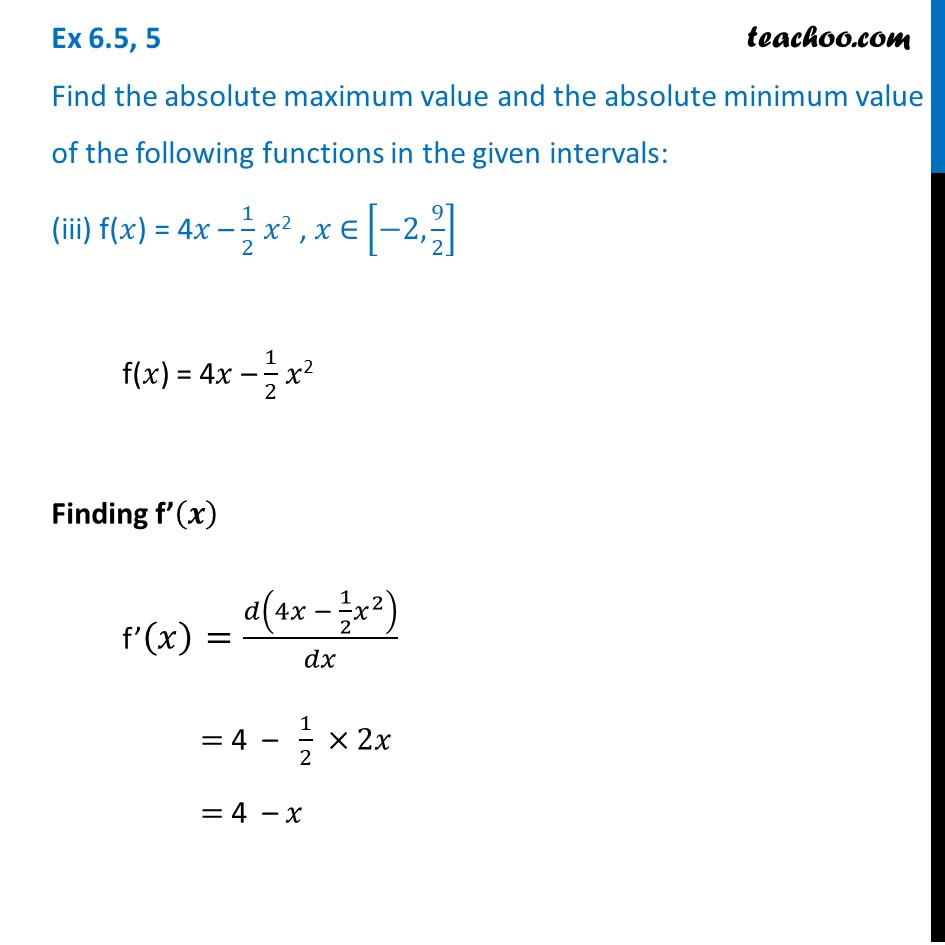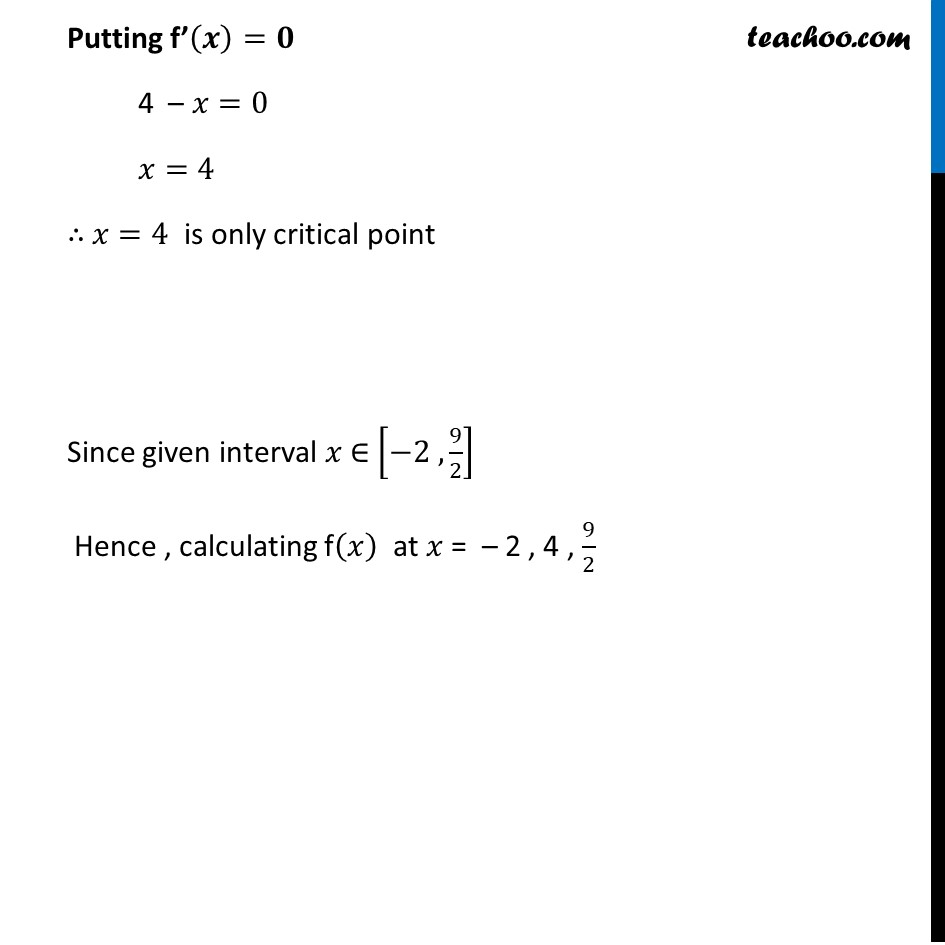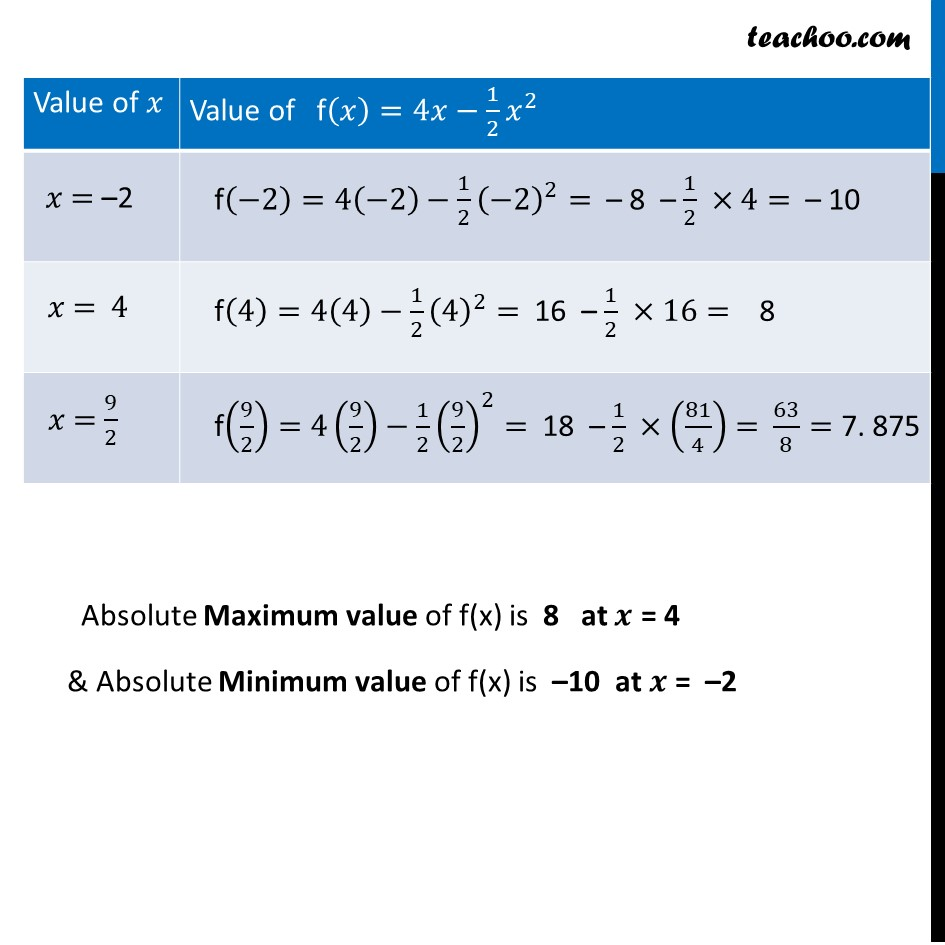Ex 6.3

Chapter 6 Class 12 Application of Derivatives
Serial order wiseLearn in your speed, with individual attention - Teachoo Maths 1-on-1 Class

### Transcript

Ex 6.3, 5 Find the absolute maximum value and the absolute minimum value of the following functions in the given intervals: (iii) f(𝑥) = 4𝑥 – 1/2 𝑥2 , 𝑥 ∈ [−2, 9/2] f(𝑥) = 4𝑥 – 1/2 𝑥2 Finding f’(𝒙) f’(𝑥)=𝑑(4𝑥 − 1/2 𝑥^2 )/𝑑𝑥 = 4 – 1/2 ×2𝑥 = 4 – 𝑥 Putting f’(𝒙)=𝟎 4 – 𝑥=0 𝑥=4 ∴ 𝑥=4 is only critical point Since given interval 𝑥 ∈ [−2 , 9/2] Hence , calculating f(𝑥) at 𝑥 = – 2 , 4 , 9/2 Absolute Maximum value of f(x) is 8 at 𝒙 = 4 & Absolute Minimum value of f(x) is –10 at 𝒙 = –2